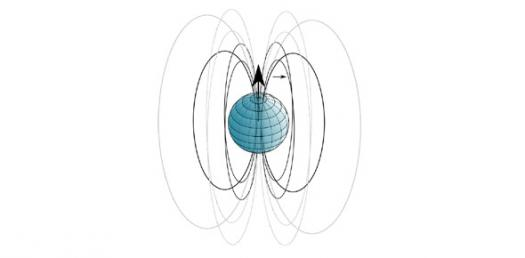# Physics Quiz: Trivia Questions On Magnetic Field

7 Questions | Total Attempts: 2009Settings.

• 1.
• A.

Electric Currents

• B.

Gravity

• C.

Friction

• D.

Heat

• 2.
Electric currents are measured in...?
• A.

Nanoteslas

• B.

Amperes

• C.

Microteslas

• D.

Newtons

• 3.
Magnetic fields move in a [blank] path.
• 4.
Lines signify the strength of magnetic fields. If the lines are denser the magnetic field is...?
• A.

Weaker

• B.

Slower

• C.

Normal

• D.

Stronger

• 5.
Magnetic forces [blank] and [blank] each other
• 6.
What causes magnetism?
• A.

Charged electric particles

• B.

Friction

• C.

Gravity

• D.

Earth's atmosphere

Related TopicsBack to top